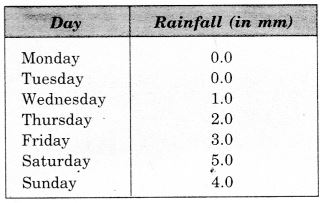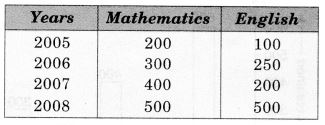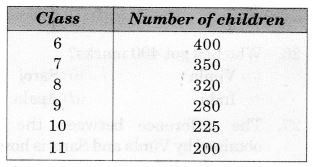# CBSEtips.in

## Thursday, 25 February 2021

### CBSE Class 7 Maths - MCQ and Online Tests - Unit 3 - Data Handling

#### CBSE Class 7 Maths – MCQ and Online Tests – Unit 3 – Data Handling

Every year CBSE students attend Annual Assessment exams for 6,7,8,9,11th standards. These exams are very competitive to all the students. So our website provides online tests for all the 6,7,8,9,11th standards’ subjects. These tests are also very effective and useful for those who preparing for any competitive exams like Olympiad etc. It can boost their preparation level and confidence level by attempting these chapter wise online tests.

These online tests are based on latest CBSE syllabus. While attempting these, our students can identify their weak lessons and continuously practice those lessons for attaining high marks. It also helps to revise the NCERT textbooks thoroughly

#### CBSE Class 7 Maths – MCQ and Online Tests – Unit 3 – Data Handling

The number of tourists visiting a historical place in a week is shown in the following table :Answer the following related questions.

Question 1.
The difference between the maximum and minimum number of tourists is
(a) 50
(b) 80
(c) 90
(d) 100

Hint:
160 – 60 = 100.

Question 2.
On which day 60 tourists visit?
(a) Monday
(b) Tuesday
(c) Friday
(d) Sunday

Hint:
60 ? Friday.

Question 3.
What is the difference between the number of tourists visiting on Friday and Monday?
(a) 10
(b) 24
(c) 38
(d) 5

Hint:
65 – 60 = 5.

Question 4.
The sum of the number of tourists visiting on Sunday and Friday is
(a) 160
(b) 60
(c) 220
(d) 100

Hint:
160 + 60 = 220.

Question 5.
On which day is the number of tourists maximum?
(a) Sunday
(b) Wednesday
(c) Tuesday
(d) Saturday

Hint:
Sunday ? 160.

Question 6.
A batsman scored the following number of runs in six innings:
35, 30, 45, 65, 39, 20
The mean runs scored by him in an inning is
(a) 39
(b) 38
(c) 37
(d) 40

Hint:
Mean = $$\frac { 35+30+45+65+39+20 }{ 6 }$$
= $$\frac { 234 }{ 6 }$$ = 39

Question 7.
The mean of the numbers 10,20, 30 and 40 is
(a) 20
(b) 25
(c) 30
(d) 50

Hint:
Mean = $$\frac { 10+20+30+40 }{ 4 }$$ = $$\frac { 100 }{ 4 }$$ = 25.

Question 8.
The range of the weights (in kg) of a students of a class given below is:
49, 60, 47, 50, 47, 59, 58, 45, 53
(a) 10
(b) 15
(c) 20
(d) 2

Hint:
Range = 60 – 45 = 15.

Question 9.
The marks of 11 students of a class are as given below:
78, 11, 99, 63, 94, 6, 78, 36, 30, 55, 22
The range of marks is
(a) 90
(b) 91
(c) 92
(d) 93

Hint:
Range = 99 – 6 = 93.

Question 10.
On which day is the number of tourists minimum?
(a) Friday
(b) Monday
(c) Thursday
(d) Saturday

Hint:
Friday ? 60.

Question 11.
The rainfall (in mm) in a city on 7 days of a certain week was recorded as follows:On how many days was the rainfall less than 6 mm?
(a) 0
(b) 3
(c) 6
(d) 7

Hint:
On each day,rainfall < 6 mm.

Question 12.
Which of the following statements is true?
(a) The mode is always one of the numbers in a data
(b) The mean is always one of the numbers in a data
(c) Mean < Mode in a data
(d) Median < Mode in a data

Answer: (a) The mode is always one of the numbers in a data

Question 13.
The marks of some students are as given below:
30, 31, 32, 32, 33, 32, 34, 35, 30, 31, 33, 32
Find the mode of their marks.
(a) 30
(b) 31
(c) 32
(d) 33

Hint:
32 occurs maximum number (4) times.

Question 14.
The median of the distribution 2, 3, 4, 7, 5, 1, 6 is
(a) 1
(b) 2
(c) 3
(d) 4

Hint:
1, 2, 3, 4, 5, 6, 7.

Question 15.
The median of the data 20, 30, 40, 10, 15, 25, 35 is
(a) 20
(b) 25
(c) 30
(d) 40

Hint:
10, 15, 20, 25, 30, 35, 40.

Question 16.
The mode of the distribution 3,5, 7, 4, 2, 1, 4, 3, 4 is
(a) 7
(b) 4
(c) 3
(d) 1

Hint:
4 occurs maximum number (3) times.

Question 17.
A die is thrown. What is the probability of getting 6?
(a) 0
(b) $$\frac { 1 }{ 6 }$$
(c) $$\frac { 1 }{ 2 }$$
(d) 1

Answer: (b) $$\frac { 1 }{ 6 }$$
Hint:
A die has six faces.

Question 18.
A coin is tossed. What is the probability of getting head?
(a) 0
(b) 1
(c) $$\frac { 1 }{ 2 }$$
(d) 2

Answer: (c) $$\frac { 1 }{ 2 }$$
Hint:
A coin has two faces.

Question 19.
A die is thrown. What is the probability of getting 1?
(a) 0
(b) 1
(c) $$\frac { 1 }{ 2 }$$
(d) $$\frac { 1 }{ 6 }$$

Answer: (d) $$\frac { 1 }{ 6 }$$
Hint:
A die has six faces.

Question 20.
A coin is tossed. What is the probability of getting tail?
(a) 1
(b) $$\frac { 1 }{ 2 }$$
(c) 2
(d) 0

Answer: (b) $$\frac { 1 }{ 2 }$$
Hint:
A coin has two faces.

Read the following bar graph and answer the following related questions :Question 21.
Who has got 400 marks?
(a) Vimla
(b) Saroj
(c) Indu
(d) Apala

Hint:
400 ? Vimla.

Question 22.
Who got the minimum marks?
(a) Apala
(b) Meenu
(c) Vimla
(d) Indu

Hint:
Meenu ? 100.

Question 23.
The difference between maximum and minimum marks is
(a) 100
(d) 200
(c) 400
(d) 500

Hint:
600 – 100 = 500.

Question 24.
The ratio between the marks obtained by Saroj and Vimla is
(a) 1 : 2
(b) 2 : 3
(c) 3 : 4
(d) 1 : 6

Answer: (a) 1 : 2
Hint:
200 : 400 = 1 : 2.

Question 25.
How many girls have got marks more than 100?
(a) 2
(b) 3
(c) 4
(d) 1

Hint:
200, 300, 400, 500 ? 4.

Question 26.
Who got the maximum marks?
(a) Apala
(b) Meenu
(c) Saroj
(d) Indu

Hint:
Apala ? 600.

Question 27.
The difference between the marks obtained by Vimla and Saroj is how many times the difference between the marks obtained by Meenu and Saroj?
(a) 2
(b) 3
(c) 4
(d) 6

Hint:
400 – 200 = 200; 200 – 100 = 100; 200 = 2 x 100.

Question 28.
How many girls have got marks less than 600?
(a) 1
(b) 2
(c) 3
(d) 4

Hint:
100, 200, 300, 400 ? 4.

Sale of Mathematics and English books in the year 2005, 2006, 2007 and 2008 are given below :Answer the following related questions :

Question 29.
The rise in the sale of Mathematics books from 2005 to 2008 is
(a) 100
(b) 200
(c) 300
(d) 400

Hint:
500 – 200 = 300.

Question 30.
In which year is the difference in the sale maximum?
(a)2005
(b) 2006
(c) 2007
(d) 2008

Hint:
400 – 200 = 200.

Question 31.
The ratio of sales in the year 2005 is
(a) 2 : 1
(b) 3 : 1
(c) 4 : 1
(d) 2 : 3

Answer: (a) 2 : 1
Hint:
200 : 100 = 2 : 1.

Question 32.
In which year is the difference in the sale minimum?
(a) 2008
(b) 2007
(c) 2006
(d) 2005

Hint:
2008 ? 500 – 500 = 0.

Question 33.
The fall in the sale of English books from 2006 to 2007 is
(a) 50
(b) 100
(c) 150
(d) 200

Hint:
250 – 200 = 50.

Number of children in six different classes are given below :Answer the following related questions.

Question 34.
In how many classes is the number of children more than 100?
(a) 6
(b) 4
(c) 3
(d) 1

Hint:
In each class, number of students > 100.

Question 35.
In which class is the number of children minimum?
(a) 8
(b) 9
(c) 10
(d) 11

Hint:
11 ? 200.

Question 36.
The difference between the maximum and minimum number of children is
(a) 100
(b) 200
(c) 300
(d) 400

Hint:
400 – 200 = 200.

Question 37.
In how many classes is the number of children less than 500?
(a) 2
(b) 4
(c) 5
(d) 6

Hint:
In each class, number of students < 500.

Question 38.
The ratio of the number of children of class 6 and 11 is
(a) 3 : 1
(b) 2 : 1
(c) 2 : 3
(d) 1 : 4

Answer: (b) 2 : 1
Hint:
400 : 200 = 2 : 1.

Question 39.
The total number of children is
(a) 1775
(b) 1675
(c) 1575
(d) 1785

Hint:
400 + 350 + 320 + 280 + 225 + 200 = 1775.

Question 40.
In which class is the number of children maximum?
(a) 6
(b) 7
(c) 8
(d) 9

Hint:
6 ? 400.

Share: